Published in Comput. Methods Appl. Mech. Engrg. Vol. 195, pp. 6750-6777, 2006
doi: 10.1016/j.cma.2004.10.018

Abstract

The paper describes some recent developments in finite element and particle methods for analysis of a wide range of bulk forming processes. The developments include new stabilized linear triangles and tetrahedra using finite calculus and a new procedure combining particle methods and finite element methods. Applications of the new numerical methods to casting, forging and other bulk metal forming problems and mixing processes are shown.

Keywords: Bulk forming processes, stabilized finite element method, particle method, particle finite element method, mixing processes.

1 INTRODUCTION

The development of efficient and robust numerical methods for analysis of bulk forming problems has been a subject of intensive research in recent years [1-7]. Many of these problems require the solution of incompressible fluid flow situations (such as in mould filling problems) whereas in other cases (such as forging, rolling, extrusion, etc.) the numerical method must be able to account for the quasi/fully incompressible behaviour induced by the large plastic deformation. The solution of these problems has motivated the development of the so called stabilized numerical methods overcoming the two main sources of instability in the analysis of incompressible continua, namely those originated by the high values of the convective terms in fluid flow situtions and those induced by the difficulty in satisfying the incompressibility condition.

Different approaches to solve both type of problems in the context of the finite element method (FEM) have been recently developed . Traditionally, the underdiffusive character of the Galerkin FEM for high convection flows has been corrected by adding some kind of artificial viscosity terms to the standard Galerkin equations [8,9].

A popular way to overcome the problems with the incompressibility constraint in the FEM is by introducing a pseudo-compressibility in the continuum and using implicit and explicit algorithms ad hoc such as artificial compressibility schemes  and preconditioning techniques . Other FEM schemes with good stabilization properties for the convective and incompressibility terms in fluid flows are based in Petrov-Galerkin (PG) techniques. The background of PG methods are the non-centred (upwind) schemes for computing the first derivatives of the convective operator in FD and FV methods [8,9,12]. A general class of Galerkin FEM has been developed where the standard Galerkin variational form is extended with adequate residual-based terms in order to achieve a stabilized numerical scheme. Among the many FEM of this kind we can name the Streamline Upwind Petrov Galerkin (SUPG) method [8,13-16], the Galerkin Least Square (GLS) method [8,17], the Taylor-Galerkin method , the Characteristic Galerkin method  and its variant the Characteristic Based Split (CBS) method [20,21], the pressure gradient operator method  and the Subgrid Scale (SS) method . A good review of these methods can be found in . Extensions of the CBS and SS methods to treat incompressible problems in solid mechanics are reported in [25,26,58] and [27-29], respectively.

In this paper a different class of stabilized FEM for quasi and fully incompressible fluid and solid materials applicable to a wide range of bulk forming problems is presented. The starting point is the modified governing differential equations of the continuous problem formulated via a finite calculus (FIC) approach [30,31]. The FIC method is based in invoking the classical balance (or equilibrium) laws in a domain of finite size. This introduces naturally additional terms in the differential equations of infinitesimal continuum mechanics which are a function of the balance domain dimensions. The new terms in the modified governing equations provide the necessary stabilization to the discrete equations obtained via the standard Galerkin FEM. One of the main advantages of the FIC formulation versus other alternative approaches (such as mixed FEM, etc.) is that it allows to solve incompressible fluid problems using low order finite elements (such as linear triangles and tetrahedra) with equal order approximations for the velocity and pressure variables [32-35]. The FIC formulation has been successfully used for analysis of fully or quasi incompressible solids [36,37].

The layout of the paper is the following. In the next section the basic FIC equations for incompressible flow problems formulated in an Eulerian frame are presented. The finite element discretization is introduced and the resulting discretized equations are detailed. A fractional step scheme for the transient solution is presented.

The stabilized Eulerian formulation is extended to account for thermal effects and the transport of the free surface which are needed for mould filling processes.

The following sections outline the FIC formulation for analysis of quasi/fully incompressible solids using a Lagrangian description and linear triangles and tetrahedra. An explicit algorithm for integrating in time the equations of motion of elasto-plastic solids in large-strain problems involving frictional contact is described. Examples of application to a casting problem and some bulk forming processes are presented.

In the last part of the paper a Lagrangian formulation for fluid flow analysis is presented as a straightforward extension of the formulation for solid mechanics. The procedure, called the Particle Finite Element Method (PFEM) [38-40], treats the mesh nodes in the fluid and solid domains as dimensionless particles which can freely move, an even separate from the main fluid domain, representing, for instance, the effect of liquid drops. A finite element mesh connects the nodes defining the discretized domain where the governing equations are solved in the standard FEM fashion. The main advantage of the Lagrangian flow formulation is that the convective terms do not enter in the fluid equations. The difficulty is however transferred to the problem of adequately (and efficiently) moving the mesh nodes. The final examples show that the PFEM is a promising method to solve mould filling and casting problems, material mixing processes and many other bulk metal forming problems involving the interaction between solids and fluids which can be treated with the same formulation.

2 FIC EQUATIONS FOR VISCOUS INCOMPRESSIBLE FLOWS

The FIC governing equations for a viscous incompressible fluid can be written in an Eulerian frame of reference as [30-34]

Momentum

 $r_{m_{i}}-{\underline {{1 \over 2}h_{j}{\partial r_{m_{i}} \over \partial x_{j}}}}{1 \over 2}h_{j}{\partial r_{m_{i}} \over \partial x_{j}}=0\quad {\hbox{in }}\Omega$
(1)

Mass balance

 $r_{d}-{\underline {{1 \over 2}h_{j}{\partial r_{d} \over \partial x_{j}}}}{1 \over 2}h_{j}{\partial r_{d} \over \partial x_{j}}=0\quad {\hbox{in }}\Omega$
(2)

where

 $r_{m_{i}}=\rho \left({\partial v_{i} \over \partial t}+v_{j}{\partial v_{i} \over \partial x_{j}}\right)+{\partial p \over \partial x_{i}}-{\partial s_{ij} \over \partial x_{j}}-b_{i}$ (3) $r_{d}={\partial v_{i} \over \partial x_{i}}\qquad i,j=1,n_{d}$ (4)

Above ${\textstyle \Omega }$ is the analysis domain which can evolve with time, ${\textstyle n_{d}}$ is the number of space dimensions (${\textstyle n_{d}=3}$ for 3D problems), ${\textstyle v_{i}}$ is the velocity along the ith global axis, ${\textstyle \rho }$ is the (constant) density of the fluid, ${\textstyle p}$ is the absolute pressure (defined positive in compression), ${\textstyle b_{i}}$ are the body forces and ${\textstyle s_{ij}}$ are the deviatoric stresses related to the viscosity ${\textstyle \mu }$ by the standard expression

 $s_{ij}=2\mu \left({\dot {\varepsilon }}_{ij}-\delta _{ij}{1 \over 3}{\partial v_{k} \over \partial x_{k}}\right)$
(5)

where ${\textstyle \delta _{ij}}$ is the Kronecker delta and the strain rates ${\textstyle {\dot {\varepsilon }}_{ij}}$ are

 ${\dot {\varepsilon }}_{ij}={1 \over 2}\left({\partial v_{i} \over \partial x_{j}}+{\partial v_{j} \over \partial x_{i}}\right)$
(6)

The boundary conditions are written in the FIC approach as

 $n_{j}\sigma _{ij}-t_{i}^{p}+{\underline {{1 \over 2}h_{j}n_{j}r_{m_{i}}}}{1 \over 2}h_{j}n_{j}r_{m_{i}}=0\quad {\hbox{on }}\Gamma _{t}$
(7)
 $v_{j}-v_{j}^{p}=0\quad {\hbox{on }}\Gamma _{u}$
(8)

and the initial condition is ${\textstyle v_{j}=v_{j}^{0}}$ for ${\textstyle t=t_{0}}$.

Summation convention for repeated indexes in products and derivatives is used unless otherwise specified.

In Eqs.(7) and (8) ${\textstyle t_{i}^{p}}$ and ${\textstyle v_{j}^{p}}$ are surface tractions and prescribed velocities on the boundaries ${\textstyle \Gamma _{t}}$ and ${\textstyle \Gamma _{u}}$, respectively, ${\textstyle n_{j}}$ are the components of the unit normal vector to the boundary and ${\textstyle \sigma _{ij}}$ are the total stresses given by ${\textstyle \sigma _{ij}=s_{ij}-\delta _{ij}p}$.

Eqs. (1) and (2) are obtained by invoking the classical balance equations in fluid mechanics in a domain of finite size and retaining higher order terms . The ${\textstyle h_{i}'s}$ in above equations are characteristic lengths of the domain where the balance of momentum and mass is enforced. In Eq.(7) these lengths define the domain where equilibrium of boundary tractions is established. In the discretized problem the ${\textstyle h_{i}}$ coincide with a typical element dimension, as described in Section 5. Note that by making ${\textstyle h_{i}=0}$ in these equations the standard infinitesimal form of the fluid mechanics equations is recovered [8,9,24].

Eqs.(1)–(8) are the starting point for deriving stabilized FEM for solving the incompressible Navier-Stokes equations using equal order interpolation for the velocity and pressure variables [32-35]. Application of the FIC formulation to meshless analysis of fluid flow problems using the finite point method can be found in .

Remark. In most metal forming processes the viscosity ${\textstyle \mu }$ is a non linear function of the strain rate and the yield stress of the material (which also depends on the temperature) [8,43,44]. This dependence adds another non linearity to the problem.

Remark. In Eqs.(1)–(7) ${\textstyle \Omega }$ and ${\textstyle \Gamma _{t}}$ respectively denote the actual volume and the boundary of the domain where the governing equations are solved at each instant of the forming processes. This domain is considered here to be fixed in space (Eulerian approach). Moving free surfaces, such as in the case of mold filling processes, can be modelled by using standard level set and volume of fluid (VOF) techniques [47,48]. An alternative is to use an arbitrary Lagragian-Eulerian (ALE) description or even a fully Lagrangian description to follow the motion of the particles during the forming process. The Lagrangian description is typical in the case of solid mechanics problems (Section 5). A particular Lagrangian formulation for fluid flow problems involving large motion of the free surface, named the Particle Finite Element Method, is presented in Section 8.

2.1 Stabilized integral forms

From the momentum equations it can be obtained [32-34]

 ${\partial r_{d} \over \partial x_{i}}\simeq {h_{j} \over 2a_{i}}{\partial r_{m_{i}} \over \partial x_{j}}\quad ,\quad {\hbox{no sum in }}i$
(9)

where

 $a_{i}={2\mu \over 3}+{\rho v_{i}h_{i} \over 2}\quad ,\quad {\hbox{no sum in }}i$
(10)

Substituting Eq.(9) into Eq.(2) and retaining the terms involving the derivatives of ${\textstyle r_{m_{i}}}$ with respect to ${\textstyle x_{i}}$ only, leads to the following expression for the stabilized mass balance equation

 $r_{d}-\sum \limits _{i=1}^{n_{d}}\tau _{i}{\partial r_{m_{i}} \over \partial x_{i}}=0$
(11)

with

 $\tau _{i}=\left({8\mu \over 3h_{i}^{2}}+{2\rho v_{i} \over h_{i}}\right)^{-1}$
(12)

The ${\textstyle \tau _{i}}$'s in Eq.(12), when scaled by the density, are termed in the stabilization literature intrinsic time parameters.

The weighted residual form of the momentum and mass balance equations (Eqs.(1) and (11)) is written as

 $\int _{\Omega }\delta v_{i}\left[r_{m_{i}}-{h_{j} \over 2}{\partial r_{m_{i}} \over \partial x_{j}}\right]d\Omega +\int _{\Gamma _{t}}\delta v_{i}(\sigma _{ij}n_{j}-t_{i}^{p}+{h_{j} \over 2}n_{j}r_{m_{i}})d\Gamma =0$
(13)
 $\int _{\Omega }q\left[r_{d}-\sum \limits _{i=1}^{n_{d}}\tau _{i}{\partial r_{m_{i}} \over \partial x_{i}}\right]d\Omega =0$
(14)

where ${\textstyle \delta v_{i}}$ and ${\textstyle q}$ are arbitrary weighting functions representing virtual velocities and virtual pressure fields. Integrating by parts the ${\textstyle r_{m_{i}}}$ terms leads to

 $\int _{\Omega }\delta v_{i}r_{m_{i}}d\Omega +\int _{\Gamma _{t}}\delta v_{i}(\sigma _{ij}n_{j}-t_{i})d\Gamma +\int _{\Omega }{h_{j} \over 2}{\partial \delta v_{i} \over \partial x_{j}}r_{m_{i}}d\Omega =0$
(15a)
 $\int _{\Omega }qr_{d}d\Omega +\int _{\Omega }\left[\sum \limits _{i=1}^{n_{d}}\tau _{i}{\partial q \over \partial x_{i}}r_{m_{i}}\right]d\Omega -\int _{\Gamma }\left[\sum \limits _{i=1}^{n_{d}}q\tau _{i}n_{i}r_{m_{i}}\right]d\Gamma =0$
(15b)

We will neglect hereonwards the third integral in Eq.(15b) by assuming that ${\textstyle r_{m_{i}}}$ is negligible on the boundaries. The deviatoric stresses and the pressure terms in the first integral of Eq.(15a) are integrated by parts in the usual manner. The resulting momentum and mass balance equations are

 ${\begin{array}{r}\displaystyle \int _{\Omega }\left[\delta v_{i}\rho \left({\partial v_{i} \over \partial t}+v_{j}{\partial {v_{i}} \over \partial x_{j}}\right)+{\partial \delta v_{i} \over \partial x_{j}}\left(\mu {\partial v_{i} \over \partial x_{j}}-\delta _{ij}p\right)\right]d\Omega -\int _{\Omega }\delta v_{i}b_{i}d\Omega -\int _{\Gamma _{t}}\delta v_{i}t_{i}^{p}d\Gamma +\qquad \\\displaystyle +\int _{\Omega }{h_{j} \over 2}{\partial \delta v_{i} \over \partial x_{j}}r_{m_{i}}d\Omega =0\qquad \end{array}}$
(16a)
 $\int _{\Omega }q{\partial v_{i} \over \partial x_{i}}d\Omega +\int _{\Omega }\left[\sum \limits _{i=1}^{n_{d}}\tau _{i}{\partial q \over \partial x_{i}}r_{m_{i}}\right]d\Omega =0$
(16b)

In the derivation of the viscous term in Eq.(16a) we have used the following identity (prior to the integration by parts)

 ${\partial s_{ij} \over \partial x_{j}}=2\mu {\partial \varepsilon _{ij} \over \partial x_{j}}=\mu {\partial ^{2}v_{i} \over \partial x_{j}\partial x_{j}}$
(17)

Eq.(17) is identically true for the exact incompressible limit ${\textstyle \left({\partial v_{i} \over \partial x_{i}}=0\right)}$.

2.2 Convective and pressure gradient projections

The computation of the residual terms can be simplified if we introduce now the convective and pressure gradient projections ${\textstyle c_{i}}$ and ${\textstyle \pi _{i}}$, respectively defined as

 ${\begin{array}{l}\displaystyle c_{i}=r_{m_{i}}-\rho v_{j}{\partial v_{i} \over \partial x_{j}}\\\displaystyle \pi _{i}=r_{m_{i}}-{\partial p \over \partial x_{i}}\end{array}}$
(18)

We can express ${\textstyle r_{m_{i}}}$ in Eqs.(16a) and (16b) in terms of ${\textstyle c_{i}}$ and ${\textstyle \pi _{i}}$, respectively which then become additional variables. The system of integral equations is now augmented in the necessary number of equations by imposing that the residual ${\textstyle r_{m_{i}}}$ vanishes (in average sense) for both forms given by Eqs.(18). This gives the final system of governing equation as:

 $\int _{\Omega }\left[\delta v_{i}\rho \left({\partial v_{i} \over \partial t}+v_{j}{\partial {v_{i}} \over \partial x_{j}}\right)+{\partial \delta v_{i} \over \partial x_{j}}\left(\mu {\partial v_{i} \over \partial x_{j}}-\delta _{ij}p\right)\right]d\Omega -\int _{\Omega }\delta v_{i}b_{i}d\Omega -\int _{\Gamma _{t}}\delta v_{i}t_{i}^{p}d\Gamma +$ $+\int _{\Omega }{h_{k} \over 2}{\partial (\delta v_{i}) \over \partial x_{k}}\left(\rho v_{j}{\partial {v_{i}} \over \partial x_{j}}+c_{i}\right)d\Omega =0\qquad$
(19)
 $\int _{\Omega }q{\partial v_{i} \over \partial x_{i}}d\Omega +\int _{\Omega }\sum \limits _{i=1}^{n_{d}}\tau _{i}{\partial q \over \partial x_{i}}\left({\partial p \over \partial x_{i}}+\pi _{i}\right)d\Omega =0$
(20)
 $\int _{\Omega }\delta c_{i}\rho \left(\rho v_{j}{\partial {v_{i}} \over \partial x_{j}}+c_{i}\right)d\Omega =0\qquad {\hbox{no sum in }}i$
(21)
 $\int _{\Omega }\delta \pi _{i}\tau _{i}\left({\partial p \over \partial x_{i}}+\pi _{i}\right)d\Omega =0\qquad {\hbox{no sum in }}i$
(22)

with ${\textstyle i,j,k=1,n_{d}}$. In Eqs.(21) and (22) ${\textstyle \delta c_{i}}$ and ${\textstyle \delta \pi _{i}}$ are appropriate weighting functions and the ${\textstyle \rho }$ and ${\textstyle \tau _{i}}$ weights are introduced for convenience.

We note that accounting for the convective and pressure gradient projections enforces the consistency of the formulation as it ensures that the stabilization terms in Eqs.(19–22) have a residual form which vanishes for the “exact” solution. Neglecting these terms lowers the accuracy of the numerical solution and it makes the formulation more sensitive to the value of the stabilization parameters as shown in [34,36,37].

3 FINITE ELEMENT DISCRETIZATION

We choose ${\textstyle C^{\circ }}$ continuous linear interpolations of the velocities, the pressure, the convection projections ${\textstyle c_{i}}$ and the pressure gradient projections ${\textstyle \pi _{i}}$ over three node triangles (2D) and four node tetrahedra (3D) . The linear interpolations are written as

 ${\begin{array}{l}\displaystyle v_{i}=N^{k}{\bar {v}}_{i}^{k}\quad ,\quad p=N^{k}{\bar {p}}^{k}\\\displaystyle c_{i}=N^{k}{\bar {c}}_{i}^{k}\quad ,\quad \pi _{i}=N^{k}{\bar {\pi }}_{i}^{k}\end{array}}$
(23)

where the sum goes over the number of nodes of each element ${\textstyle n}$ (${\textstyle n=3/4}$ for triangles/tetrahedra), ${\textstyle {\bar {(\cdot )}}^{k}}$ denotes nodal variables and ${\textstyle N^{k}}$ are the linear shape functions .

Substituting the approximations (23) into Eqs.(19–22) and choosing the Galerking form with ${\textstyle \delta v_{i}=q=\delta c_{i}=\delta \pi _{i}=N^{i}}$ leads to following system of discretized equations

 $\displaystyle {\bf {M}}{\dot {\bar {\bf {v}}}}+{\bf {H}}{\bar {\bf {v}}}-{\bf {G}}{\bar {\bf {p}}}+{\bf {C}}{\bar {\bf {c}}}={\bf {f}}$
(24a)
 $\displaystyle {\bf {G}}^{T}{\bar {\bf {v}}}+{\hat {\bf {L}}}{\bar {\bf {p}}}+{\bf {Q}}{\bar {\boldsymbol {\pi }}}={\bf {0}}$
(24b)
 $\displaystyle {\hat {\bf {C}}}{\bar {\bf {v}}}+{\bf {M}}{\bar {\bf {c}}}={\bf {0}}$
(24c)
 $\displaystyle {\bf {Q}}^{T}{\bar {\bf {p}}}+{\hat {\bf {M}}}{\bar {\boldsymbol {\pi }}}={\bf {0}}$
(24d)

The form of the different matrices is given in the Appendix.

The solution in time of the system of Eqs.(24) can be written in general form as

 ${\bf {M}}\displaystyle {1 \over \Delta t}({\bar {\bf {v}}}^{n+1}-{\bar {\bf {v}}}^{n})+{\bf {H}}^{n+\theta }{\bar {\bf {v}}}^{n+\theta }-{\bf {G}}{\bar {\bf {p}}}^{n+\theta }+{\bf {C}}^{n+\theta }{\bar {\bf {c}}}^{n+\theta }={\bf {f}}^{n+\theta }$
(25a)
 ${\bf {G}}^{T}{\bar {\bf {v}}}^{n+\theta }+{\hat {\bf {L}}}^{n+\theta }{\bar {\bf {p}}}^{n+\theta }+{\bf {Q}}{\bar {\boldsymbol {\pi }}}^{n+\theta }={\bf {0}}$
(25b)
 ${\hat {\bf {C}}}^{n+\theta }{\bar {\bf {v}}}^{n+\theta }+{\bf {M}}{\bar {\bf {c}}}^{n+\theta }={\bf {0}}$
(25c)
 ${\bf {G}}^{T}{\bar {\bf {p}}}^{n+\theta }+{\hat {\bf {M}}}^{n+\theta }{\bar {\boldsymbol {\pi }}}^{n+\theta }={\bf {0}}$
(25d)

where ${\textstyle {\bf {H}}^{n+\theta }={\bf {H}}({\bar {\bf {v}}}^{n+\theta })}$, ${\textstyle {\bar {\bf {v}}}^{n+\theta }}$ are the velocities evaluated at time ${\textstyle n+\theta }$ and the parameter ${\textstyle \theta \in [0,1]}$. The direct monolitic solution of Eqs.(25) is possible using an adequate iterative scheme. However, we have found more convenient to use a fractional step method as described in the next section.

3.1 Fractional step method

A fractional step scheme is derived by noting that the discretized momentum equation (25a) can be split into the two following equations

 ${\bf {M}}\displaystyle {1 \over \Delta t}({\tilde {\bf {v}}}^{n+1}-{\bar {\bf {v}}}^{n})+{\bf {H}}^{n+\theta }{\bar {\bf {v}}}^{n+\theta }-\alpha {\bf {G}}{\bar {\bf {p}}}^{n}+{\bf {C}}^{n+\theta }{\bar {\bf {c}}}^{n+\theta }={\bf {f}}^{n+\theta }$
(26)
 ${\bf {M}}\displaystyle {1 \over \Delta t}({\bar {\bf {v}}}^{n+1}-{\tilde {\bf {v}}}^{n+1})-{\bf {G}}({\bar {\bf {p}}}^{n+1}-\alpha {\bar {\bf {p}}}^{n})={\bf {0}}$
(27)

In above equation ${\textstyle {\tilde {v}}^{n+1}}$ is a predicted value of the velocity at time ${\textstyle n+1}$ and ${\textstyle \alpha }$ is a variable whose values of interest are zero and one. For ${\textstyle \alpha =0}$ (first order scheme) the splitting error is of order ${\textstyle 0(\Delta t)}$, whereas for ${\textstyle \alpha =1}$ (second order scheme) the error is of order ${\textstyle 0(\Delta t^{2})}$ .

Eqs.(26) and (27) are completed with the following three equations emanating from Eqs.(25b-d)

 ${\bf {G}}^{T}{\bar {\bf {v}}}^{n+1}+{\hat {\bf {L}}}^{n}{\bar {\bf {p}}}^{n+1}+{\bf {Q}}{\bar {\boldsymbol {\pi }}}^{n}={\bf {0}}$
(28a)
 ${\hat {\bf {C}}}^{n+1}{\bar {\bf {v}}}^{n+1}+{\bf {M}}{\bar {\bf {c}}}^{n+1}={\bf {0}}$
(28b)
 ${\bf {Q}}^{T}{\bar {\bf {p}}}^{n+1}+{\hat {\bf {M}}}^{n+1}{\bar {\boldsymbol {\pi }}}^{n+1}={\bf {0}}$
(28c)

The value of ${\textstyle {\bar {\bf {v}}}^{n+1}}$ obtained from Eq.(27) is substituted into Eq.(28a) to give

 ${\bf {G}}^{T}{\tilde {\bf {v}}}^{n+1}+\Delta t{\bf {G}}^{T}{\bf {M}}^{-1}{\bf {G}}({\bar {\bf {p}}}^{n+1}-\alpha {\bar {\bf {p}}}^{n})+{\hat {\bf {L}}}^{n}{\bf {p}}^{n+1}+{\bf {Q}}{\bar {\boldsymbol {\pi }}}^{n}={\bf {0}}$
(29)

The product ${\textstyle {\bf {G}}^{T}{\bf {M}}^{-1}{\bf {G}}}$ can be approximated by a laplacian matrix, i.e.

 ${\bf {G}}^{T}{\bf {M}}^{-1}{\bf {G}}={1 \over \rho }{L}\quad {\hbox{with }}L^{ab}=\int _{\Omega ^{e}}{\boldsymbol {\nabla }}^{T}N^{a}{\boldsymbol {\nabla }}N^{b}d\Omega$
(30)

where ${\textstyle L^{ab}}$ are the element contributions to ${\textstyle {L}}$ (see Appendix).

The steps of the fractional step scheme chosen here are:

Step 1

The fractional nodal velocities ${\textstyle {\tilde {v}}^{n+1}}$ can be explicitely computed from Eq.(26) by

 ${\tilde {\bf {v}}}^{n+1}={\bar {\bf {v}}}^{n}-\Delta t{\bf {M}}_{d}^{-1}[{\tilde {\bf {H}}}^{n}{\bar {\bf {v}}}^{n}-\alpha {\bf {G}}{\bar {\bf {p}}}^{n}+{\bf {C}}^{n}{\bar {\bf {c}}}^{n}-{\bf {f}}^{n}]$
(31)

where ${\textstyle {\bf {M}}_{d}}$ is the diagonal form of ${\textstyle {\bf {M}}}$ obtaining by lumping the row terms into the corresponding diagonal terms.

Step 2 Compute ${\textstyle {\bar {\bf {p}}}^{n+1}}$ from Eq.(29) as

 ${\bar {\bf {p}}}^{n+1}=-[{\hat {\bf {L}}}^{n}+{\Delta t \over \rho }{\bf {L}}]^{-1}[{\bf {G}}^{T}{\tilde {\bf {v}}}^{n+1}-\alpha {\Delta t \over \rho }{\bf {L}}{\bar {\bf {p}}}^{n}+{\bf {Q}}{\bar {\boldsymbol {\pi }}}^{n}]$
(32)

Step 3 Compute ${\textstyle {\bar {\bf {v}}}^{n+1}}$ explicitely from Eq.(28a) as

 ${\bar {\bf {v}}}^{n+1}={\tilde {\bf {v}}}^{n+1}+\Delta t{\bf {M}}_{d}^{-1}{\bf {G}}({\bar {\bf {p}}}^{n+1}-\alpha {\bar {\bf {p}}}^{n})$
(33)

Step 4 Compute ${\textstyle {\bar {\bf {c}}}^{n+1}}$ explicitely from Eq.(28b) as

 ${\bar {\bf {c}}}^{n+1}=-{\bf {M}}_{d}^{-1}{\hat {\bf {C}}}^{n+1}{\bar {\bf {v}}}^{n+1}$
(34)

Step 5 Compute ${\textstyle {\bar {\boldsymbol {\pi }}}^{n+1}}$ explicitely from Eq.(28c) as

 ${\bar {\boldsymbol {\pi }}}^{n+1}=-{\hat {\bf {M}}}_{d}^{-1}{\bf {Q}}^{T}{\bar {\bf {p}}}^{n+1}$
(35)

where ${\textstyle {\hat {\bf {M}}}_{d}}$ is the lumped form of ${\textstyle {\hat {\bf {M}}}}$. A standard diagonal lumping procedure based in summing up the terms of each row has been used.

Note that all steps can be solved explicitely except for the computation of the pressure in Eq.(32) which requires to invert the sum of two laplacian matrices. This can be effectively performed using an iterative solution scheme such as the conjugate gradient method.

Above algorithm has improved stabilization properties versus the standard segregation methods due to the introduction of the laplacian matrix ${\textstyle {\hat {\bf {L}}}}$ in Eq.(32). This matrix emanates from the FIC formulation.

The boundary conditions are applied as follows. No condition is applied in the computation of the fractional velocities ${\textstyle {\tilde {\bf {v}}}^{n+1}}$ in Eq.(31). The prescribed velocities at the boundary are applied when solving for ${\textstyle {\bar {\bf {v}}}^{n+1}}$ in step 3. The prescribed pressures at the boundary are imposed by making ${\textstyle {\bar {\bf {p}}}^{n}}$ equal to the prescribed pressure values when solving Eq.(32).

3.2 Stokes flow

Many metal forming processes can be simulated with the assumption that the convective terms are negligible (Stokes flow) [8,43,44]. The Stokes FIC formulation can be readily obtained simply by neglecting the convective terms in the Navier-Stokes formulation presented earlier. This also implies neglecting the convective stabilization terms in the momentum equations and, consequently, the convective projection variables are not larger necessary. Also the intrinsic time parameters ${\textstyle \tau _{i}}$ take now the simpler form (see Eq.(12)):

 $\tau _{i}={3h_{i}^{2} \over 8\mu }$
(36)

We note again that in metal forming problems the viscosity ${\textstyle \mu }$ will typically be a function of the strain rate and the yield stress of the material 8.

The resulting discretized system of equations can be written as (see Eqs.(28))

 ${\begin{array}{l}\displaystyle {\bf {M}}{\dot {\bar {\bf {v}}}}+{\bf {K}}{\bar {\bf {v}}}-{\bf {G}}{\bar {\bf {p}}}={\bf {f}}\\\displaystyle {\bf {G}}^{T}{\bar {\bf {v}}}+{\hat {\bf {L}}}{\bar {\bf {p}}}+{\bf {Q}}{\bar {\boldsymbol {\pi }}}={\bf {0}}\\\displaystyle {\bf {Q}}^{T}{\bar {\bf {p}}}+{\hat {\bf {M}}}{\bar {\boldsymbol {\pi }}}={\bf {0}}\end{array}}$
(37)

The algorithm of previous section can now be implemented.

The steady-state form of Eqs.(37) can be expressed in matrix form as

 $\left[{\begin{matrix}{\bf {K}}&-{\bf {G}}&{\bf {0}}\\-{\bf {G}}^{T}&-{\hat {\bf {L}}}&-{\bf {Q}}\\{\bf {0}}&-{\bf {Q}}^{T}&-{\hat {\bf {M}}}\\\end{matrix}}\right]\left\{{\begin{matrix}{\bar {\bf {v}}}\\{\bar {\bf {p}}}\\{\bar {\boldsymbol {\pi }}}\\\end{matrix}}\right\}=\left\{{\begin{matrix}{\bf {f}}\\{\bf {0}}\\{\bf {0}}\\\end{matrix}}\right\}$
(38)

The system is symmetric and always positive definite and therefore leads to a non singular solution. This property holds for any interpolation function chosen for ${\textstyle {\bar {\bf {v}}},{\bar {\bf {p}}}}$ and ${\textstyle {\bar {\boldsymbol {\pi }}}}$, therefore overcoming the Babuŝka-Brezzi (BB) restrictions .

A reduced velocity-pressure formulation can be obtained by elliminating the ${\textstyle {\bar {\pi }}}$ variables from the last row of Eq.(38) .

The FIC formulation for Stokes flows is applicable for analysis of quasi/fully incompressible solids. An analogous formulation based on solid mechanics concepts is derived in Section 6.

4 THERMAL-COUPLED FLOWS. TREATMENT OF THE FREE BOUNDARY

4.1 Thermal coupled flow

The effect of temperature can be easily introduced by solving the equation for heat transport coupled to the fluid flow equations.

The equation of balance of heat is written in the FIC formulation as 

 $r_{\phi }-{h_{j} \over 2}{\partial r_{\phi } \over \partial x_{j}}=0\qquad ,\quad j=1,n_{d}$
(39a)

with

 $r_{\phi }:=-\rho c\left({\partial \phi \over \partial t}+v_{i}{\partial \phi \over \partial x_{i}}\right)+{\partial \over \partial x_{k}}\left(k{\partial \phi \over \partial x_{k}}\right)+Q$
(39b)

In above ${\textstyle \phi }$ is the temperature, ${\textstyle c}$ and ${\textstyle k}$ are the specific heat and the thermal conductivity of the material, respectively, ${\textstyle Q}$ is the heat source and ${\textstyle h_{j}}$ are the characteristic length distances which are typical of the FIC formulation .

Eq.(39a) is completed with the Dirichlet and Neuman boundary conditions for the heat problem. For details see .

The convective velocities ${\textstyle v_{i}}$ in Eq.(39b) are provided by the solution of the fluid flow problem. As usual in metal forming processes, the heat source ${\textstyle Q}$ is a function of the mechanical work generated in the flow of the material during the forming process. The temperature field affects in turn the flow viscosity via its dependence with the yield stress which is very sensitive to the temperature changes. The solution of the heat transfer equation is therefore fully coupled with that of the fluid flow problem [30,46].

4.2 Treatment of the free boundary motion

For the treatment of the free boundary we use a standard volume of fluid (VOF) technique, also known as pseudo-concentration method or level set technique [22,47,48]. In the VOF method the motion of the free boudary is followed by solving the following transport equation (written using the FIC formulation)

 $r_{\beta }-{\underline {{{\bar {h}}_{j} \over 2}{\partial r_{\beta } \over \partial x_{j}}}}{{\bar {h}}_{j} \over 2}{\partial r_{\beta } \over \partial x_{j}}=0\quad {\hbox{in }}\Omega$
(40a)

where

 $r_{\beta }:={\partial \beta \over \partial t}+v_{k}{\partial \beta \over \partial x_{k}}$
(40b)

In above ${\textstyle \beta }$ is an auxiliary variable which takes a value equal to one on the free surface and zero elsewhere. The solution of Eq.(40a) in time in the discretized fluid-air domain allows to track the free surface which is characterized by the contours of ${\textstyle \beta }$ which have a unit value.

The underlined term in Eq.(40a) emerges from the FIC formulation . Again, the ${\textstyle {\bar {h}}_{j}}$'s in Eq.(40a) are characteristic length distances of the order of the element size. This term introduces the necessary stabilization in the transient solution of Eq.(40a).

4.3 Fully coupled algorithm for mould filling problems

The analysis of mould filling problems typically requires the solution of the coupled-thermal flow problem accounting for the transport of the free surface.

The simplest explicit algorithm is as follows:

• For each time step:
• Compute the velocities and the pressure in the fluid ${\textstyle ({\bar {v}}_{i}^{n+1},{\bar {p}}^{n+1})}$ using the fractional step scheme of Section 3.1.
• Compute the nodal temperatures ${\textstyle (\phi ^{n+1})}$ solving Eq.(39a).
• Compute the new position of the free surface ${\textstyle (\beta ^{n+1})}$ solving Eq.(40a).

A number of implicit versions of the algorithm are possible and they all involve an iteration loop within each time step until convergence for the flow variables, the temperature and the free surface position is found. For details see [47,48].

5 COMPUTATION OF THE CHARACTERISTIC LENGTHS

The evaluation of the stabilization parameters is one of the crucial issues in stabilized methods. Excellent results have been obtained in all problems solved using linear tetrahedra with the characteristic length vector defined by

 ${\bf {h}}=h_{s}{{\bf {v}} \over {v}}+h_{c}{{\boldsymbol {\nabla }}v \over \vert {\boldsymbol {\nabla }}v\vert }$
(41a)

where ${\textstyle v=\vert {\bf {v}}\vert }$ and ${\textstyle h_{s}}$ and ${\textstyle h_{c}}$ are the “streamline” and “cross wind” contributions given by

 $h_{s}=\max({l}_{j}^{T}{\bf {v}})/{v}$
(41b)
 $h_{c}=\max({\bf {l}}_{j}^{T}{\boldsymbol {\nabla }}v)/\vert {\boldsymbol {\nabla }}v\vert \quad ,\quad j=1,n_{s}$
(41c)

where ${\textstyle {\bf {l}}_{j}}$ are the vectors defining the element sides (${\textstyle n_{s}=6}$ for tetrahedra).

As for the free surface equation the following value of the characteristic length vector ${\textstyle {\bar {\bf {h}}}}$ in Eq.(40a) has been taken

 ${\bar {\bf {h}}}={\bar {h}}_{s}{{\bf {v}} \over {v}}+{\bar {h}}_{c}{{\boldsymbol {\nabla }}\beta \over \vert {\boldsymbol {\nabla }}\beta \vert }$
(42a)

The streamline parameter ${\textstyle {\bar {h}}_{s}}$ has been obtained by Eq.(41b) whereas the cross wind parameter ${\textstyle {\bar {h}}_{c}}$ has been computed by

 ${\bar {h}}_{c}=\max[{\bf {l}}_{j}^{T}{\boldsymbol {\nabla }}\beta ]{1 \over \vert {\boldsymbol {\nabla }}\beta \vert }\quad ,\quad j=1,2,3$
(42b)

The cross-wind terms in eqs.(41a) and (42a) account for the effect of the gradient of the solution in the stabilization parameters. This is a standard assumption in most “shock-capturing” stabilization procedures [8,24].

6 FIC FORMULATION FOR QUASI/FULLY INCOMPRESSIBLE SOLIDS

6.1 Equilibrium equations

As usual the governing equations of solid mechanics are written in a Lagrangian reference frame. Following the arguments of Section 2 the equilibrium equations for a solid are written using the FIC technique as 

 ${r}_{i}-{\frac {{h}_{k}}{2}}{\partial {r}_{i} \over \partial {x}_{k}}=0\quad {\mbox{in}}\;\,\Omega \qquad k=1,n_{d}$
(43)

where for the dynamic case

 ${r}_{i}:=-\rho {\partial ^{2}u_{i} \over \partial t^{2}}+{\partial {\sigma }_{ij} \over \partial {x}_{j}}+{b}_{i}\qquad ,\quad j=1,n_{d}$
(44)

In Eqs.(43) and (44) ${\textstyle u_{i}}$ are the displacements, ${\sigma }_{ij}$and ${b}_{i}$are the stresses and the body forces, respectively and ${h}_{k}$are characteristic length distances of an arbitrary prismatic domain where equilibrium of forces is considered.

Equations (43) and (44) are completed with the boundary conditions on the displacements ${\textstyle u_{i}}$

 ${u}_{i}-{u}_{i}^{p}=0\quad {\mbox{on}}\;\,\Gamma _{u}$
(45)

and the equilibrium of surface tractions

 ${\sigma }_{ij}{n}_{j}-t_{i}^{p}-{\underline {{\frac {1}{2}}{h}_{k}{n}_{k}{r}_{i}}}{\frac {1}{2}}{h}_{k}{n}_{k}{r}_{i}=0\quad {\mbox{on}}\;\,\Gamma _{t}$
(46)

In the above ${\textstyle {u}_{i}^{p}}$ and ${\textstyle t_{i}}$ are prescribed displacements and tractions over the boundaries ${\textstyle \Gamma _{u}}$and ${\textstyle \Gamma _{t}}$, respectively and ${\textstyle n_{i}}$ are the components of the unit normal vector.

Note that for consistency with the fluid flow equations of previous section and differently from the tradition in solid mechanics, the compression pressure has been taken as positive.

6.2 Stabilized pressure constitutive equations

For simplicity the treatment of the constitutive equations will be explained for the linear elastic model. The approach extends naturally to the non linear elasto-plastic/viscoplastic constitutive equations typical of metal forming problems.

As usual in quasi-incompressible problems the stresses are split into deviatoric and volumetric (pressure) parts

 ${\sigma }_{ij}=s_{ij}-p{\delta }_{ij}$
(47)

where ${\delta }_{ij}$is the Kronnecker delta function. The linear elastic constitutive equations for the deviatoric stresses ${\textstyle s_{ij}}$ are written as

 $s_{ij}\!\!=\!\!2G\left({\varepsilon }_{ij}-{\frac {1}{3}}{\varepsilon }_{v}{\delta }_{ij}\right)$
(48)

where ${\textstyle G}$is the shear modulus,

 ${\varepsilon }_{ij}={\frac {1}{2}}\left({\partial {u}_{i} \over \partial {x}_{j}}+{\partial {u}_{j} \over \partial x_{i}}\right)\quad {\mbox{and}}\quad {\varepsilon }_{v}={\varepsilon }_{ii}\,.$
(49)

The constitutive equation for the pressure ${\textstyle p}$ can be written for an arbitrary domain of finite size of volume ${\textstyle V}$ as

 ${1 \over K}p^{av}=-{\Delta V \over V}$
(50)

where ${\textstyle K}$ is the bulk modulus of the material and ${\textstyle p^{av}}$ is the average value of the pressure over domain ${\textstyle V}$.

The value of ${\textstyle p^{av}}$ can be approximated as 

 $p^{av}:={1 \over V}\int _{V}p\,dV=p-{h_{k} \over 2}{\partial p \over \partial x_{k}}+O(h_{k})^{2}\quad ,\quad k=1,n_{d}$
(51)

where ${\textstyle p}$ is the pressure at an arbitrary point within the domain ${\textstyle V}$ and ${\textstyle h_{k}}$ are characteristic lengths of such a domain.

The ratio ${\textstyle {\Delta V \over V}}$ can be expressed as

 ${\Delta V \over V}={\varepsilon }_{v}-{h_{k} \over 2}{\partial {\varepsilon }_{v} \over \partial x_{k}}+O(h_{k})^{2}\quad ,\quad k=1,n_{d}$
(52)

Substituting Eqs.(51) and (52) into Eq.(50) and neglecting second order terms in ${\textstyle h_{k}}$ gives the FIC constitutive equation for the pressure as

 $\left({p \over K}+{\varepsilon }_{v}\right)-{\frac {{h}_{k}}{2}}{\partial \over \partial {x}_{k}}\left({p \over K}+{\varepsilon }_{v}\right)=0\,,\quad k=1,n_{d}$
(53)

Note that for ${\textstyle h_{k}\to 0}$ the standard relationship between the pressure and the volumetric strain of the infinitesimal theory ${\textstyle (p=-K\varepsilon _{v})}$ is found.

For an incompressible material ${\textstyle K\to \infty }$ and Eq.(53) yields

 $\varepsilon _{v}-{\frac {{h}_{k}}{2}}{\partial {\varepsilon }_{v} \over \partial {x}_{k}}=0$
(54)

Eq.(54) expresses the limit incompressible behaviour of the solid. This equation is identical to Eq.(2) for incompressible flow problems and there arises from the mass continuity condition [30,32].

By combining Eqs. (43), (44), (47), (48) and (53) a mixed displacement–pressure formulation can be written as

 $-\rho {\partial ^{2}u_{i} \over \partial t^{2}}+{\partial \sigma _{ij} \over \partial x_{i}}+{b}_{i}-{\frac {{h}_{k}}{2}}{\partial r_{i} \over \partial {x}_{k}}=0$
(55)

 $\left({\frac {p}{K}}+{\varepsilon }_{v}\right)-{\frac {{h}_{k}}{2}}{\partial \over \partial {x}_{k}}\left({\frac {p}{K}}+{\varepsilon }_{v}\right)=0$
(56)

From the observation of Eq.(55) we can obtain after some algebra 

 ${\partial \over \partial x_{k}}\left({\frac {p}{K}}+{\varepsilon }_{v}\right)\simeq {3h_{j} \over 8G}{\partial r_{k} \over \partial x_{j}}$
(57)

Substituting Eq.(57) into (53) the mass balance equation can be written as

 $\left({\frac {p}{K}}+{\varepsilon }_{v}\right)-\sum \limits _{i=1}^{n_{d}}\tau _{i}{\partial r_{i} \over \partial x_{i}}=0\quad {\hbox{with }}\quad \tau _{i}=\displaystyle {3h_{i}^{2} \over 8G}$
(58)

In the derivation of Eq.(58) we have neglected the terms involving products ${\textstyle h_{i}h_{j}}$ for ${\textstyle h_{i}\not =h_{j}}$.

The pressure gradient projections ${\textstyle \pi _{i}}$ are introduced now as

 $\pi _{i}\equiv r_{i}-{\partial p \over \partial x_{i}}$
(59)

The final system of governing equations is

 $\rho {\partial ^{2}u_{i} \over \partial t^{2}}-{\partial \sigma _{ij} \over \partial x_{i}}-{b}_{i}=0$
(60)
 ${\Delta p \over k}+{\partial (\Delta u_{i}) \over \partial x_{i}}+\sum \limits _{i=1}^{n_{d}}\tau _{i}\left({\partial p \over \partial x_{i}}+\pi _{i}\right)=0$
(61)
 $r_{i}\equiv {\partial p \over \partial x_{i}}+\pi _{i}=0$
(62)

In Eqs.(60)–(62) we note the following:

• The stabilization terms have been neglected in Eq.(60). These terms were useful to derive the stabilized form of Eq.(61). However they are not longer necessary as the convective terms do not appear in the equilibrium equations.
• The constitutive equation for the pressure (Eq.(61)) has been written in an incremental form. This is more convenient for non linear material behaviour typical of metal forming situations. Here, appropriate elasto-plastic or elasto-viscoplastic constitutive laws relating stresses and strains in incremental or rate forms must be used [1-5,43,44].

6.3 Finite element equations

The weighted residual form of the governing equations can be written as (after integration by parts of the relevant terms)

 $\int _{\Omega }\delta u_{i}\rho {\frac {\partial ^{2}{u_{i}}}{\partial {t}^{2}}}\,d\Omega +\int _{\Omega }\delta {\varepsilon }_{ij}{\sigma }_{ij}\,d\Omega {-}\int _{\Omega }\delta u_{i}b_{i}\,d\Omega -\int _{\Gamma _{t}}\delta {u}_{i}t_{i}^{p}\,d\Gamma _{t}=0$
(63a)
 $\int _{\Omega }q\left({\Delta p \over K}+{\partial (\Delta u_{i}) \over \partial x_{i}}\right)\,d\Omega +\int _{\Omega }\left[\sum \limits _{i=1}^{n_{d}}{\partial q \over \partial x_{i}}\tau _{i}\left({\partial p \over \partial x_{i}}+\pi _{i}\right)\right]d\Omega =0$
(63b)
 $\int _{\Omega }\delta \pi _{i}\tau _{i}\left({\partial p \over \partial x_{i}}+\pi _{i}\right)d\Omega =0\quad {\hbox{no sum in }}i$
(63c)

where again the ${\textstyle \tau _{i}}$ are introduced in Eq.(63c) for symmetry reasons.

The finite element discretization of the displacements, the pressure and the pressure gradient projections is written by expressions identical to Eqs.(23) with the nodal variables now being a function of the time ${\textstyle t}$. Substituting the approximations into eqs.(63) and using the Galerkin form gives the following system of discretized equations

 ${\bf {M}}{\ddot {\bar {\bf {u}}}}+{\bf {g}}-{\bf {f}}={\bf {0}}$
(64a)
 ${\bf {G}}^{T}\Delta {\bar {\bf {u}}}+{\bf {C}}\Delta {\bar {\bf {p}}}+{\bf {L}}{\bar {\bf {p}}}+{\bf {Q}}{\bar {\boldsymbol {\pi }}}={\bf {0}}$
(64b)
 ${\bf {Q}}^{T}{\bar {\bf {p}}}+{\bar {\bf {C}}}{\bar {\boldsymbol {\pi }}}={\bf {0}}$
(64c)

where ${\textstyle {\ddot {\bar {u}}}}$ is the nodal acceleration vector,

 $M_{ij}=\int _{\Omega ^{e}}\rho N_{i}N_{j}\,d\Omega$
(65)

is the mass matrix,

 ${\textbf {g}}=\int _{\Omega }{B}^{T}{\boldsymbol {\sigma }}d\Omega$
(66)

is the internal nodal force vector and the rest of matrices and vectors are defined in the Appendix. Note that the expression of ${\textstyle {g}}$ of eq.(66) is adequate for non linear analysis.

A four steps semi-explicit time integration algorithm can be derived as follows:

Step 1. Compute the nodal velocities ${\textstyle {\dot {\bar {\textbf {u}}}}^{n+1/2}}$ from Eq.(64a)

 ${\dot {\bar {\textbf {u}}}}^{n+1/2}={\dot {\bar {\textbf {u}}}}^{n-1/2}+\Delta t{\textbf {M}}_{d}^{-1}({\textbf {f}}^{n}-{\textbf {g}}^{n})$
(67a)

Step 2. Compute the nodal displacements ${\textstyle {\bar {\textbf {u}}}^{n+1}}$

 ${\bar {\textbf {u}}}^{n+1}={\bar {\textbf {u}}}^{n}+\Delta t{\dot {\bar {\textbf {u}}}}^{n+1/2}$
(67b)

Step 3. Compute the nodal pressures ${\textstyle {\bar {\textbf {p}}}^{n+1}}$ from Eq.(64b)

 ${\bar {\textbf {p}}}^{n+1}=-[{\textbf {C}}+{\textbf {L}}]^{-1}[\Delta t{\textbf {G}}^{T}{\dot {\bar {\textbf {u}}}}^{n+1/2}-{\textbf {C}}{\bar {\textbf {p}}}^{n}+{\textbf {Q}}{\bar {\boldsymbol {\pi }}}^{n}]$
(67c)

Step 4. Compute the nodal projected pressure gradients ${\textstyle {\bar {\boldsymbol {\pi }}}^{n+1}}$ from Eq.(64c)

 ${\bar {\boldsymbol {\pi }}}^{n+1}=-{\bar {\textbf {C}}}_{d}^{-1}{\textbf {Q}}^{T}{\bar {\textbf {p}}}^{n+1}$
(67d)

In above, all matrices are evaluated at ${\textstyle t^{n+1}}$, ${\textstyle (\cdot )_{d}}$ denotes a lumped diagonal matrix and

 ${\textbf {g}}^{n}=\int _{\Omega ^{e}}[{B}^{T}{\boldsymbol {\sigma }}]^{n}\,d\Omega$
(68)

where the stresses ${\textstyle {\boldsymbol {\sigma }}^{n}}$ are obtained by consistent integration of the adequate (non linear) constitutive law.

Note that steps 1, 2 and 4 are fully explicit as a diagonal form of matrices ${\textstyle {\textbf {M}}}$ and ${\textstyle {\bar {\textbf {C}}}}$ has been chosen. The solution of step 3 requires invariably the inverse of a Laplacian matrix. This can be an inexpensive process using an iterative equation solution method (e.g. a preconditioned conjugate gradient method).

For the full incompressible case ${\textstyle K=\infty }$ and ${\textstyle {\textbf {C}}=0}$ in all above equations.

The critical time step ${\textstyle \Delta t}$ is taken as that of the standard explicit dynamic scheme (see Section 6.5 and [9,37]).

Fully explicit algorithm

A fully explicit four steps algorithm can be obtained by computing ${\textstyle {\bar {p}}^{n+1}}$ from step 3 in eq.(67c) as follows

 ${\bar {\textbf {p}}}^{n+1}=-{\textbf {C}}_{d}^{-1}[\Delta t{\textbf {G}}^{T}{\dot {\bar {\textbf {u}}}}^{n+1/2}-({\textbf {C}}_{d}-{\textbf {L}}){\bar {\textbf {p}}}^{n}+{\textbf {Q}}{\bar {\boldsymbol {\pi }}}^{n}]$
(69)

Note that the explicit algorithm is not applicable in the full incompressible limit as the solution of Eq.(69) breaks down for ${\textstyle K=\infty }$ and ${\textstyle {\textbf {C}}=0}$. The explicit form can however be used with success in problems where quasi-incompressible regions exist adjacent to standard “compressible” zones. Examples of this kind are shown in the next section. In both cases the semi-explicit and fully explicit schemes gave identical results with important savings in both computer time and memory storage requirements obtained when using the explicit form.

6.4 Thermal coupled effects

The effect of temperature can be easily accounted for by solving the heat transfer equation formulated in a Lagrangian frame as

 $\rho c{\partial \phi \over \partial t}-{\partial \over \partial x_{j}}\left(k{\partial \phi \over \partial x_{j}}\right)-Q=0$
(70)

As usual, the source ${\textstyle Q}$ is dependent of the mechanical work generated during the forming process.

Note that the convective terms do not enter into Eq.(70) This also eliminates the need to stabilize the numerical solution.

The coupled thermal-mechanical problem requires the computation of the temperature ${\textstyle \phi }$ at each time step using a transient solution scheme [47,48,50].

Remark. Above formulation is similar to that developed by Chiumenti et al. [27,29] and Cervera et al.  for analysis of incompressible problems in solid mechanics using a sub-grid scale approach.

6.5 Computation of the intrinsic time parameter for quasi/fully incompressible solids

In solid mechanics applications it is usual to accept that all ${\textstyle \tau _{i}}$ are identical and constant within each element and given by

 $\tau ^{(e)}={3(h^{(e)})^{2} \over 8G}\quad {\hbox{with}}\quad h^{(e)}=[V^{(e)}]^{1/n_{d}}\,T$
(71)

where ${\textstyle V^{(e)}}$ is the element volume (or the element area for 2D problems). This expression for ${\textstyle \tau ^{(e)}}$ does not take into account the element distorsions along a particular direction during the deformation process.

The correct value of the shear modulus in the expression of ${\textstyle \tau ^{(e)}}$ is another sensitive issue as, obviously, for non linear problems the value of ${\textstyle G}$ will differ from the elastic modulus. This fact has been identified by Cervera et al.  for non linear analysis of incompressible problems using linear triangles.

A useful alternative to compute ${\textstyle \tau ^{(e)}}$ for explicit non linear transient situations is to make use of the value of the speed of sound in an elastic solid, defined by

 $c={\sqrt {E \over \rho }}$
(72)

where ${\textstyle E}$ is the Young modulus. The stability condition for explicit dynamic computations is given by the Courant condition defined as 

 $\Delta t^{(e)}\leq \Delta t_{c}^{(e)}={h^{(e)} \over c}$
(73)

where ${\textstyle \Delta t_{c}^{(e)}}$ is the critical time step for the element.

Accepting that ${\textstyle G\simeq {E \over 3}}$ for the incompressible case and using Eqs.(72) and (73) (assuming the identity in Eq.(73)) an alternative expression for the element intrinsic time parameter in terms of the critical time step can be found as

 $\tau ^{(e)}={[\Delta t_{c}^{(e)}]^{2} \over \rho }$
(74)

Eq.(74) shows clearly that the intrinsic time parameter varies across the mesh as a function of the critical time step for each element.

7 APPLICATIONS TO CASTING AND BULK METAL FORMING PROCESSES

7.1 Aluminium casting simulation

A numerical simulation of an aluminium casting process is presented as a demonstration of the accuracy of the stabilized formulation. The computations are performed with the finite element code VULCAN where the stabilized FEM presented has been implemented .

The analysis simulates the casting process of an aluminium (AlSi7Mg) specimen in a steel (X40CrMoV5) mould. Material behavior of aluminium casting has been modeled by a fully coupled thermo-viscoplastic model, while the steel mould has been modeled by a simpler thermo-elastic model . Geometrical and material data were provided by the foundry RUFFINI. Figure 1 shows the finite element mesh used for the part and the cooling system. The full mesh, including the mould has 380.000 four node tetrahedra. The pouring temperature is 650${\textstyle ^{\circ }}$C. Initial temperature for the mould is obtained through a thermal die-cycling simulation. Figure 2 shows the evolution of the mould temperature after 6 cycles. The cooling system has been kept at 20${\textstyle ^{\circ }}$C. Filling evolution has been simulated as in a pressure die-casting process using the stabilized VOF technique described in [47,48]. Figure 3 shows different time steps of the simulation.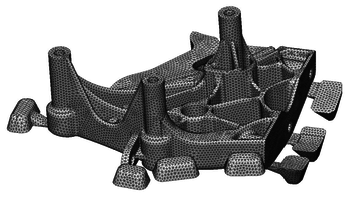Figure 1: Finite element discretization of the aluminium casting.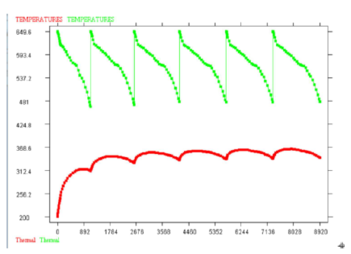Figure 2: Temperature die-cycling.

The final temperature field obtained after the filling simulation is taken as the initial condition for the solidification and cooling analysis. Temperature and liquid-fraction distributions during solidification are shown in Figures 4 and 5, respectively. The heat transfer coefficient takes into account the air-gap resistance due to the casting shrinkage during the solidification process. Figure 6 shows volumetric and von Mises deviatoric stress distributions in a x-y section. The figures also show the air-gap between the part and the mould, responsible of a non-uniform heat flux at the contact interface.

Other examples of application of the stabilized formulation to casting problems can be found in [47-51].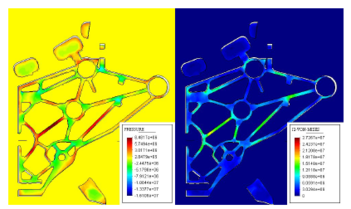Figure 6: Aluminium casting. Stress-trace and von Mises deviatoric stress indicator during phase-change (plane xy).

7.2 Side pressing of a cylinder

A cylinder 100 mm long with a radius of 100 mm is subjected to sidepressing between two plane dies. It is compressed to 100 mm. The material properties are the following: ${\textstyle E=217}$ GPa, ${\textstyle \nu =0.3}$, ${\textstyle \rho =7830}$ kg/m${\textstyle ^{3}}$, ${\textstyle \sigma _{0}=170}$ MPa, ${\textstyle H=30}$ MPa, friction coefficient = 0.2. The die velocity is assumed to be 2 m/s. Initial set-up is shown in Figure 7. A quarter of a cylinder was discretized with tri-linear hexahedra and linear tetrahedra meshes.

Figure 8 shows the results obtained using the hexahedral mesh and a standard mixed formulation. The results show the distribution of the effective plastic strain and pressure on the deformed shape. The sensitivity of the FIC results with the expression of the intrinsic time parameter of Eq.(71) was studied by defining ${\textstyle \tau ^{(e)}=a{[h^{(e)}]^{2} \over G}}$ and solving the problem for different values of ${\textstyle a}$. The results obtained with the FIC method are shown in Figs. 9 and 10 for ${\textstyle a=0.1}$ and ${\textstyle 0.03}$, respectively. The alternative expression for ${\textstyle \tau ^{(e)}}$ of Eq.(74) has also been studied. The results for this case are shown in Fig. 11. Quite a good agreement can be seen between the FIC solutions and the reference solution with the best results for the effective plastic strain obtained for ${\textstyle \tau ^{(e)}}$ calculated according to Eq. (71) with ${\textstyle a=0.03}$. The results for the two alternative formulae for ${\textstyle \tau ^{(e)}}$ are similar, but those obtained using Eq. (71) seem to be slightly better. In any case, the results on the pressure distribution are quite insentitive to the value of ${\textstyle \tau ^{(e)}}$. This is also confirmed in Fig. 12b, which displays the distribution of the pressure along the line ${\textstyle ABCDEA}$ defined in Fig. 12a. A small perturbation can be seen at the sharp edges of the deformed body.

All the calculations here have been carried out using fully explicit version of the algorithm which is more efficient than the semi-implicit one with giving practically the same results.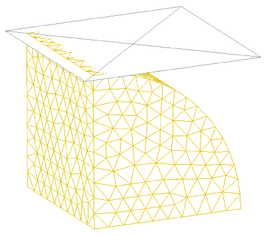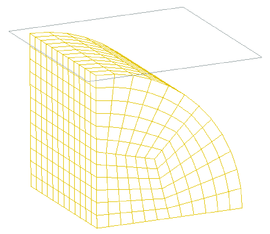Figure 7: Sidepressing of a cylinder: (a) initial tetrahedral mesh; (b) initial hexahedral mesh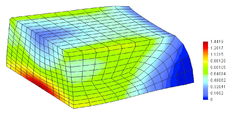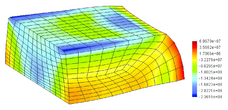Figure 8: Sidepressing of a cylinder, mixed formulation, hexahedral mesh: (a) effective plastic strain; (b) pressure distribution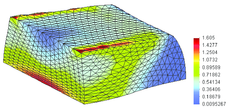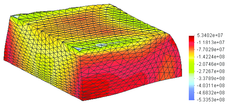Figure 9: Sidepressing of a cylinder, FIC algorithm ($\alpha =0.1$), tetrahedra, mesh of 22186 elements: (a) effective plastic strain; (b) pressure distribution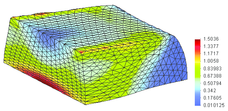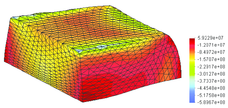Figure 10: Sidepressing of a cylinder, FIC algorithm ($a=0.03$), tetrahedra, mesh of 22186 elements: (a) effective plastic strain; (b) pressure distribution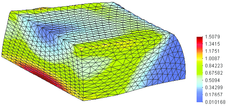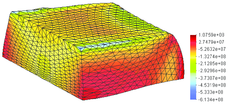Figure 11: Sidepressing of a cylinder, FIC algorithm ($\tau ^{(e)}$ calculated according to Eq. (74)), tetrahedra, mesh of 22186 elements: (a) effective plastic strain; (b) pressure distribution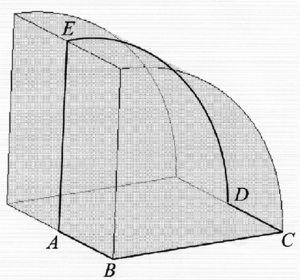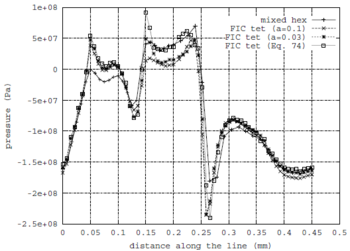Figure 12: a) Definition of the line for comparison of pressure distribution b) Pressure distribution along the line $ABCDEA$

7.3 Backward extrusion

Backward extrusion of a cylinder made of steel 16MNCr5 has been analysed using an axi-symmetric formulation. This is a benchmark example of the finite element program for forming simulation MARC/Autoforge . The tooling and billet geometry are given in Fig. 13a. Initial material dimensions are the following: length 30 mm and diameter 30 mm. The punch of diameter 20 mm has a prescribed stroke of 28 mm. Material properties are as follows: Young's modulus ${\textstyle E=3.24\cdot 10^{5}}$ MPa, Poissson's coefficient ${\textstyle \nu =0.3}$, material density ${\textstyle \rho =8120}$ kg/m${\textstyle ^{3}}$, yield stress ${\textstyle \sigma _{Y0}=300}$ MPa and hardening modulus ${\textstyle H=50}$ MPa. Friction between the material and tools is defined by the Coulomb friction coefficient ${\textstyle \mu =0.1}$.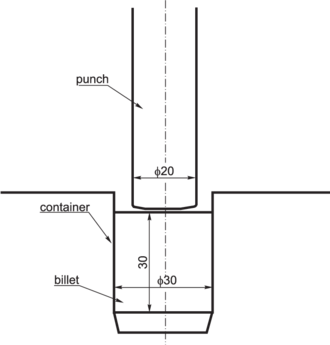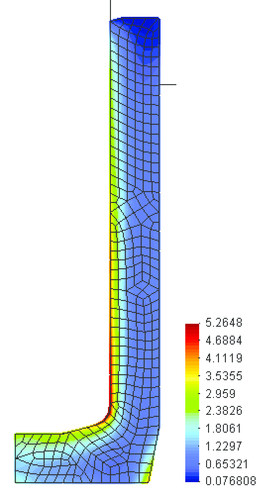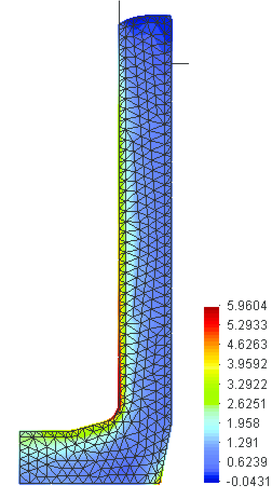(a) (b) Figure 13: Backward extrusion a) geometry definition. Final deformed shape with effective plastic strain distribution; b) solution with quadrilaterals and mixed formulation; c) solution with triangles and the FIC algorithm

The simulation of the backward extrusion process was carried out with a particularization of the FIC formulation of Section 6 for axisymmetric solids. Regeneration of the mesh was performed when element distorsion was excessive. Figures 13b and 13c show the results in the form of the final deformed shape with the distribution of the effective plastic strain obtained using quadrilaterals with a mixed formulation, and using triangles and the FIC algorithm, respectively. The results are in a good agreement with the solution given in . This example demonstrates the efficiency of the FIC algorithm for simulation of bulk forming processes. Different stages of the forming process are shown in Fig. 14.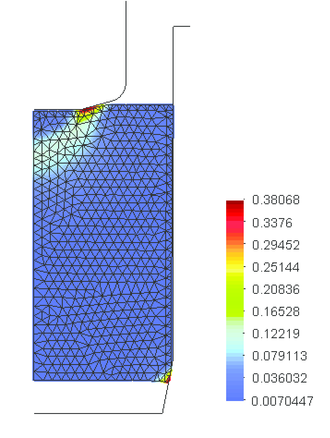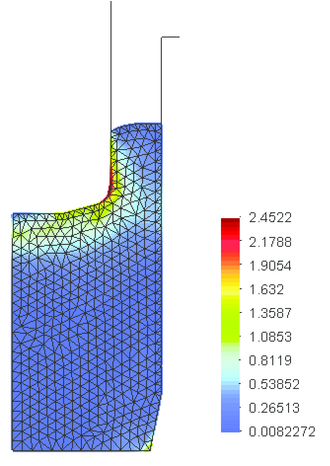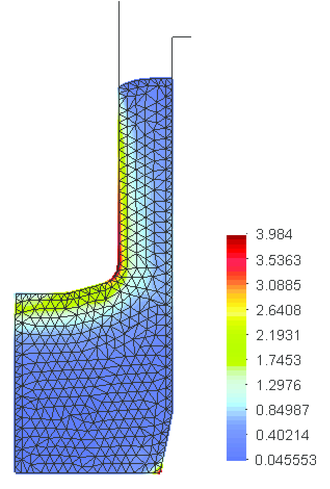Figure 14: Backward extrusion – deformed shapes with effective plastic strain distribution at different stages of forming. Solution with triangles and the FIC algorithm

7.4 Forming of a hose-clamp band

The stabilized formulation was applied to model the manufacturing of a hose-clamp band of steel AISI 409L (Fig. 15). The initial set-up of the tooling and band is shown in Fig. 16. A series of grooves are forged in the band by the roll passing over the band placed on the toothed punch. The band thickness is 0.7 mm and its width 8 mm. Plane strain conditions have been assumed. Material properties are as follows: Young's modulus ${\textstyle E=2.1\cdot 10^{5}}$ MPa, Poissson's coefficient ${\textstyle \nu =0.33}$, material density ${\textstyle \rho =7800}$ kg/m${\textstyle ^{3}}$, the true stress–true strain relationship is given by the power Ludwik–Nadai equation ${\textstyle \sigma _{Y}=623(0.36822\cdot 10^{-2}+\varepsilon _{p})^{0.1362}}$ MPa, friction between the material and tools is defined by the Coulomb friction coefficient ${\textstyle \mu =0.1}$.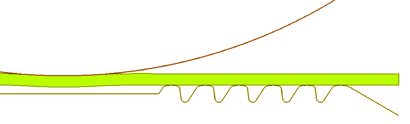Figure 16: A hose clamp – initial set-up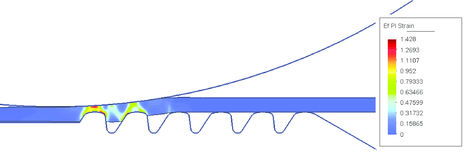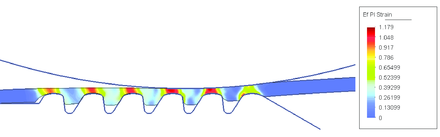Figure 17: A hose clamp – deformed shapes at different stages of forming with distribution of effective plastic strain

Simulation was carried out using linear triangular elements and the FIC formulation. As in the previous example the meshes where regenerated when element distorsion was excessive. The purpose of the simulation was to check if the expected groove depth and tooth height in the band were obtained. Figures 17a and 17b show the results in the form of the deformed shape with the distribution of the effective plastic strain at different stages of forming. The results are in good agreement with the real process. Figure 18 shows a detail of the deformed shape with finite element discretization and distribution of effective plastic strain. In this figure the obtained dimensions are compared with the required ones shown in brackets. Effects of elastic springback are also clearly seen.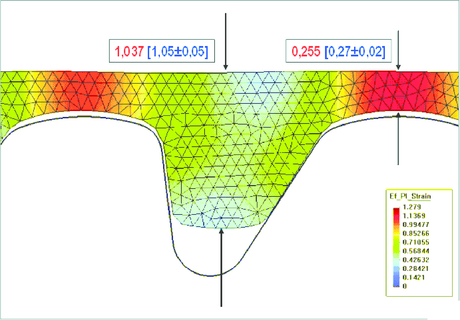Figure 18: Detail of the deformed shape with finite element discretization and distribution of effective plastic strain

Remark. The examples presented show that the FIC/FEM formulation is an effective procedure for solving bulk metal forming problems involving full or quasi-incompressible situations. The key advantage of the FIC approach versus more standard mixed FEM formulations is that it provides a natural theoretical framework for equal order finite element interpolations for the velocity and pressure variables, both in the context of implicit and explicit solution schemes. We note the simplicity and effectiveness of the full explicit algorithm as demonstrated in the examples presented. The FIC formulation reproduces also the best feature of the so called stabilized FEM method for incompressible problems, such as the CBS scheme [20,21,25,26,58], the pressure gradient operator method  and the subgrid scale method  among others.

8 LAGRANGIAN FLOWS. THE PARTICLE FINITE ELEMENT METHOD

8.1 The Particle Finite Element Method (PFEM)

The Lagrangian formulation is an excellent procedure for treating bulk forming processes involving the interaction of fluids and solids using a unified formulation. An important advantage of the Lagrangian formulation is that both the motions of the solid and the fluid are defined in the same frame of reference and modelled with the some governing equations.

The Lagrangian fluid flow equations can be simply obtained by noting that the velocity of the mesh nodes and that of the fluid particles are the same. Hence the the convective terms vanish in the momentum equations, while the rest of the fluid flow equations remain unchanged. The resulting governing equations have an identical form as those of the Stokes flow problem, with the motion of the flow particles being referred now to a Lagrangian coordinate frame.

The FEM algorithms for solving the Lagrangian flow equations are very similar to those presented for incompressible solids in a previous section. Here we present a particular class of Lagrangian formulation called the particle finite element method (PFEM) [38-41]. The PFEM treats the mesh nodes in the fluid and solid domains as dimensionless particles which can freely move an even separate from the main fluid domain representing, for instance, the effect of fluid drops. A finite element mesh connects the nodes defining the discretized domain where the governing equations are solved in the standard FEM fashion.

The quality of the numerical solution depends on the discretization chosen as in the standard FEM. Adaptive mesh refinement techniques can be used to improve the solution in zones where large motions of the fluid or the structure occur.

A typical solution with the PFEM involves the following steps.

1. Identify the external boundaries for both the fluid and solid domains. This is an essential step as some boundaries (such as the free surface in the fluid) may be severely distorted during the solution process including separation and re-entring of nodes. The Alpha Shape method is used for the boundary definition (see Section 8.4).
2. Discretize the fluid and solid domains with a finite element mesh. For the mesh generation process we use and extended Delaunay technique .
3. Solve iteratively the coupled Lagrangian equations of motion for the fluid and the solid domains. Compute the relevant state variables in both domains at each time step: velocities, pressure and viscous stresses in the fluid and displacements, stresses and strains in the solid.
4. The iterative solution scheme chosen is a particularization of the fractional step algorithm of Section 3.1 for ${\textstyle \alpha =1}$. In summary the solution steps are the following.

Step 3.1. Compute the predicted velocities (viz, Eq.(31))

 ${\tilde {\bf {v}}}^{n+1,i+1}={\bar {\bf {v}}}^{n}-\Delta t{\bf {M}}_{d}^{-1}[{\bf {K}}{\bar {\bf {v}}}^{n}-{\bf {G}}{\bar {\bf {p}}}^{n}-{\bf {f}}^{n+1}]$
(75)

Step 3.2. Compute ${\textstyle {\bar {\textbf {p}}}^{n+1,i+1}}$ from Eq.(32) for ${\textstyle \alpha =1}$.

Step 3.3. Compute explicitely ${\textstyle {\bar {\textbf {v}}}^{n+1,i+1}}$ from Eq.(33) with ${\textstyle \alpha =1}$.

Step 3.4. Compute ${\textstyle {\bar {\boldsymbol {\pi }}}^{n+1,i+1}}$ explicitely from Eq.(35).

Step 3.5. Solve for the motion of the solid. This can be performed by integrating the dynamic equations of motion in the solid. Here the algorithm of Section 6 can be used, as it applies to both “compressible” and incompressible materials.

Step 3.6. Estimate a new position of the mesh nodes in terms of the time increment size as

 ${\bf {x}}_{j}^{n+1,i+1}={\bf {x}}_{j}^{n}+{\bar {\bf {u}}}_{j}^{n+1,i+1}\Delta t$
(76)

It is important to note that all matrices in Steps 3.1–3.5 are evaluated at the last predicted configuration ${\textstyle \Omega ^{n+1,i}}$.

In steps 3.1–3.6 superindex ${\textstyle i}$ denotes the iteration within each time step.

Step 3.7. Check the convergence of the velocity and pressure fields in the fluid and the displacements, strains and stresses in the solid. If convergence is achieved froze the final position of the mesh nodes and move to the next time increment (step 4), otherwise return to step 3.1 for the next iteration.

5. Go back to step 1 and repeat the solution process for the next time step.

Above algorithm can be found to be analogous to the standard updated lagrangian scheme typically used in non linear solid mechanics problems [8,57].

Despite the motion of the nodes within the iterative process, in general there is no need to regenerate the mesh at each iteration. In the examples presented in the paper the mesh in the fluid domain has been regenerated at each time step. A cheaper alternative is to generate a new mesh only after a prescribed number of converged time steps, or when the nodal displacements induce significant geometrical distorsions in some elements.

The boundary conditions are applied as described in Section 3.1.

In the examples presented in the paper the time increment size has been chosen as

 $\Delta t=\min(\Delta t_{i})\quad {\hbox{with}}\quad \Delta t_{i}={\vert {\bf {v}}\vert \over h_{i}^{\min }}$
(77)

where ${\textstyle h_{i}^{\min }}$ is the distance between node ${\textstyle i}$ and the closest node in the mesh.

8.2 Treatment of contact between fluid and solid interfaces

The condition of prescribed velocities or pressures at the solid boundaries in the PFEM are applied in strong form to the boundary nodes. These nodes might belong to fixed external boundaries or to moving boundaries linked to the interacting solids. In some problems it is useful to define a layer of nodes adjacent to the external boundary in the fluid where the condition of prescribed velocity is imposed. These nodes typically remain fixed during the solution process. Contact between liquid particles and the solid boundaries is accounted for by the incompressibility condition which naturally prevents the liquid nodes to penetrate into the solid boundaries [38-41].

8.3 Generation of a new mesh

One of the key points for the success of the PFEM is the fast regeneration of a finite element mesh at every time step on the basis of the position of the nodes in the space domain. In our work the mesh is typically generated using the so called extended Delaunay tesselation (EDT) [38,52]. The EDT allows one to generate non standard meshes combining elements of arbitrary polyhedrical shapes (triangles, quadrilaterals and other polygons in 2D and tetrahedra, hexahedra and arbitrary polyhedra in 3D) in a computing time of order ${\textstyle n}$, where ${\textstyle n}$ is the total number of nodes in the mesh. The ${\textstyle C^{\circ }}$ continuous shape functions of the elements are obtained using the so called meshless finite element interpolation (MFEM) .

8.4 Identification of boundary surfaces

The PFEM requires the correct definition of the boundary domain. Sometimes, boundary nodes are clearly distinguished from internal nodes. In other cases, the total set of nodes is the only information available and the algorithm must recognize the boundary nodes.

Considering that the nodes follow a variable ${\textstyle h(x)}$ distribution, where ${\textstyle h(x)}$ is the minimum distance between two nodes, the following criterion has been used. For each two nodes (three nodes in 3D) a circle (a sphere in 3D) of radius equal to ${\textstyle \alpha h}$ containing the nodes is plotted. All nodes laying on a circle (sphere) with a radius greater than $\alpha h$, not containing any other node in the interior are considered as boundary nodes. In practice, ${\textstyle \alpha }$ is a parameter close to, but greater than one. This criterion coincides with the Alpha Shape concept [39,55].

The method also allows to identify isolated fluid particles (nodes) outside the main fluid domain. These particles are treated as part of the external boundary where the pressure is fixed to the atmospheric value. We note that the “flying particles” are in fact “points” with no mass which motion is followed by integrating the dynamic equations of continuum mechanics for known values of the mass, the initial velocity and acceleration, gravity body forces and a prescribed zero (atmospheric) pressure.

8.5 Temperature coupled effects

Thermal-mechanical problems can be effectively treated with the PFEM. This requires solving for the temperature field at each time step, accounting for the couplings induced by the mechanical equations. The effect of temperature in the mechanical problem is introduced via the constitutive equation in the usual manner.

The form of the heat transfer equation is identical to that of Eq.(70). Recall that the Lagrangian formulation eliminates the convective term in the heat transfer equation and hence the FIC stabilization is here not needed .

Remark

The key advantage of the PFEM versus conventional particle method is that it retains the best feature of the FEM to solve problems in continuum mechanics using a variational approach. A standard finite element mesh is used to discretize in space the problem variables and for solving the governing equations precisely as in the standard FEM. The combination of the lagrangian FEM with the Alpha-Shape approach for identification of the domain boundary at each time step and the frequent regeneration of the finite element mesh are the distinct features of the PFEM.

9 APPLICATIONS OF THE PFEM TO METAL FORMING PROCESSES

9.1 Sloshing problem

The sloshing example shown in Figure 19 ilustrates the ability of the PFEM to simulate the flow of liquids within closed cavities with large motions of the free surface. This feature of the PFEM is essential to model the filling of moulds as shown in the next examples. More applications of the PFEM to sloshing problemas are reported in .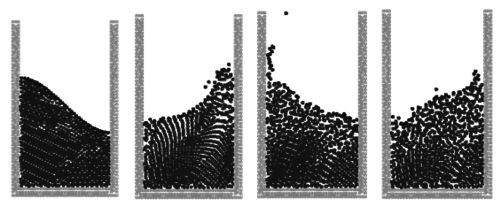Figure 19: PFEM results for a large amplitude sloshing problem

9.2 Filling of moulds

Figure 20 shows the results of the filling of a 2D mould cavity with a powder material using the PFEM. Note that the essential feature of the filling process are well reproduced. The finite element mesh used for the computation in a certain instant is shown in Figure 20b. This ilustrates the fact that the PFEM is, in fact, a blending of particle and finite element procedure. Other applications of the PFEM to powder compaction problems are reported in .

Figure 21 shows the different stages of the 3D simulation of the filling of a mould with a casted metal using the PFEM. The pictures show the evolution of the casting during the filling process. The metal flows through the vertical mould first and then fills the lower and upper lateral ducts, as expected. The gray colour intensities denote the different temperatures in the melt with a whiler colour indicating the cooler region. Note that the metal cools down as it progresses within the mould.

The example in Figure 22 shows the simulation of the casting of a mechanical part using the PFEM. Note that the mesh discretizing the casted region is progressively generated as the mould is filled. This is a distinct feature of the PFEM which distinguishes this method from more standard VOF techniques using Eulerian and ALE approaches.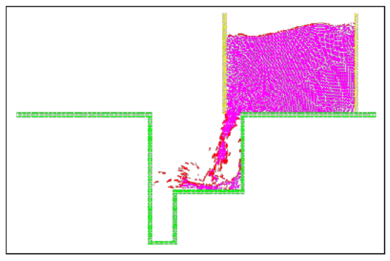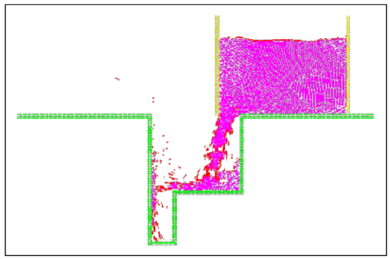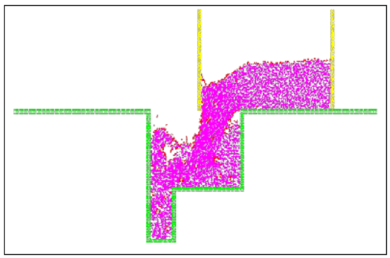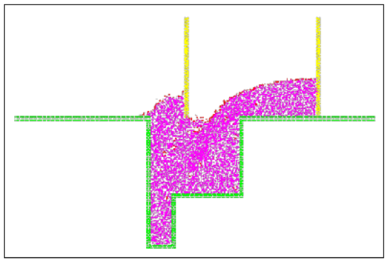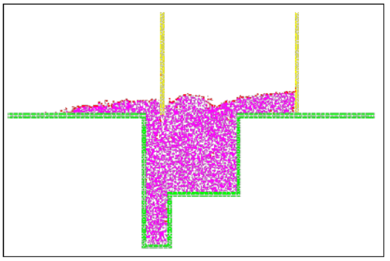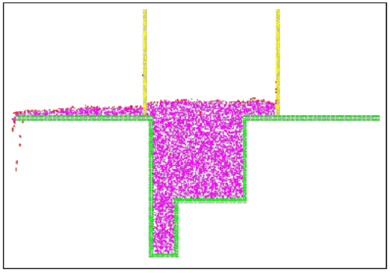(a)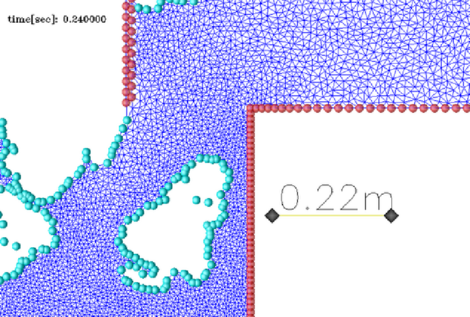(b) Figure 20: (a) Filling of a 2D mould with a powder material using the PFEM. (b) Finite element mesh used for the computations in a certain instant. The circles indicate the nodes at the external and internal free surfaces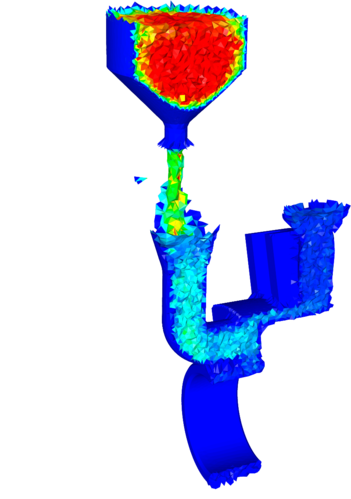Figure 22: (cont.) Cut through the mesh

9.3 Mixing problems

The PFEM is particularly suited for analyzing problems involving mixing of two fluids or that of a collection of particles in a fluid. This is very useful to model a wide class of material forming processes [5,7].

A first example of this kind is shown in Figure 23. The problem represents the mixing of two fluids of different density. The PFEM allows to track the position of the fluid particles of lower density which mix within the second fluid.

Figure 24 shows a last example of application of the PFEM to the mixing of a collection of particles within a container filled with a fluid of a higher density. Initially the particles are thrown into the container and mix within the fluid as shown. As time evolves the particles move up naturally towards the surface of the fluid due to their lower density.

The last two examples clearly show the possibilities of the PFEM for analysis of material mixing situations. Extensions of the approach accounting for thermal-coupled effects and chemical reactive situations are straightforward .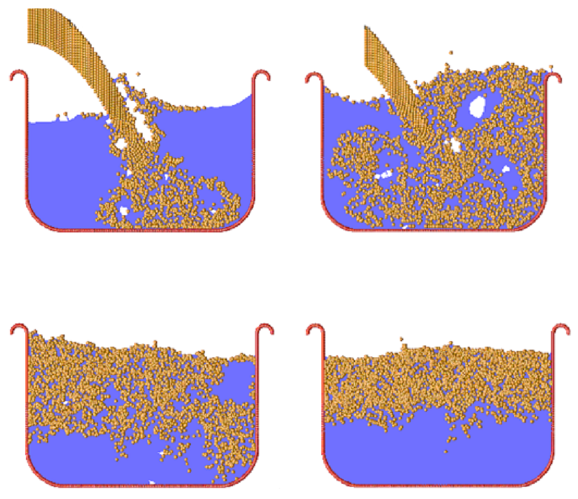Figure 24: Mixing of particles in a fluid. Evolution of the particles during the mixing process

10 CONCLUDING REMARKS

The finite calculus form of the balance equations in mechanics is a good starting point for deriving stabilized finite element methods for solving a variety of bulk metal forming problems involving fluid flow and large deformation of solids using Euler and Lagrangian descriptions. Free surface effects and coupled thermal situations can be accounted for in a straightforward manner within the general algorithm. A fractional step scheme with intrinsic stabilization properties has been described. Applications of the Eulerian and Lagrangian methods presented range from mould filling, solidification and cooling situations in casting processes to forging and extrussion problems, among many others. The Lagrangian PFEM formulation is very effective for the analysis of bulk metal forming problems and material design processes involving filling of moulds, material mixing situations and complex fluid-structure interactions.

ACKNOWLEDGEMENT

The authors thank the company QUANTECH ATZ, SA (www.quantech.es) for providing the code VULCAN for solution of the casting problem presented. Thanks are also given to MIKALOR SA for providing data for the hose clamp example.

BIBLIOGRAPHY

 J.L. Chenot and E. Oñate, Modelling of Metal Forming Processes, Kluwer Academic, Dordrecht, 1988.

 J.F.T. Pittman, O.C. Zienkiewicz, R.D. Wood and J.M. Alexander (Eds), Numerical Analysis of Forming Processes, Wiley, Chichester, 1984.

 J. Huetnik and F.P.T. Baaijens (Eds), Simulation of Materials Processing: Theory, Methods and Applications, NUMIFORM 98, Enschede, Países Bajos, A.A. Balkema, Rotterdam, 22–5 Jun. 1998.

 M. Pietrzyk, J. Kusiak, J. Majta, P. Hartley and I. Pillinger, Metal Forming 2000, A.A. Balkema, Rotterdam, 2000.

 K. Mori, Simulation of Material Processing: Theory, Methods and Applications, A.A. Balkema, Lisse, 2001.

 D.R.J. Owen, E. Oñate and B. Suarez (Eds.), Computational Plasticity VII. Fundamentals and Applications, CIMNE, Barcelona 2003.

 S. Ghosh, J.C. Castro and J.K. Lee, Material Processing and Design: Simulation and ApplicatOñate,ions, NUMIFORM 2004, American Institute of Physics, 2004.

 O.C. Zienkiewicz and R.C. Taylor,The finite element method, 5th Edition, 3 Volumes, Butterworth–Heinemann, 2000.

 C. Hirsch, Numerical computation of internal and external flow, J. Wiley, Vol. 1 1988, Vol. 2, 1990.

 J. Peraire, K. Morgan and J. Peiro, “The simulation of 3D incompressible flows using unstructured grids”, In Frontiers of Computational Fluid Dynamics, Caughey DA and Hafez MM. (eds), Chapter 16, J. Wiley, 1994.

 M. Storti, N. Nigro and S.R. Idelsohn, “Steady state incompressible flows using explicit schemes with an optimal local preconditioning”, Computer Methods in Applied Mechanics and Engineering, 124, 231–252, 1995.

 J.C. Heinrich, P.S. Hayakorn and O.C. Zienkiewicz, “An upwind finite element scheme for two dimensional convective transport equations”, Int. J. Num. Meth. Engng., 11, 131–143, 1977.

 A. Brooks and T.J.R. Hughes, “Streamline upwind/Petrov-Galerkin formulation for convection dominated flows with particular emphasis on the incompressible Navier-Stokes equations”, Comput. Methods Appl. Mech. Engrg, 32, 199–259, 1982.

 T.J.R. Hughes and M. Mallet, “A new finite element formulations for computational fluid dynamics: III. The generalized streamline operator for multidimensional advective-diffusive systems”, Comput Methods Appl. Mech. Engrg., 58, pp. 305–328, 1986.

 M.A. Cruchaga and E. Oñate, “A finite element formulation for incompressible flow problems using a generalized streamline operator”, Comput. Methods in Appl. Mech. Engrg., 143, 49–67, 1997.

 M.A. Cruchaga and E. Oñate, “A generalized streamline finite element approach for the analysis of incompressible flow problems including moving surfaces”, Comput. Methods in Appl. Mech. Engrg., 173, 241–255, 1999.

 T.J.R. Hughes, L.P. Franca and G.M. Hulbert, “A new finite element formulation for computational fluid dynamics: VIII. The Galerkin/least-squares method for advective-diffusive equations”, Comput. Methods Appl. Mech. Engrg., 73, pp. 173–189, 1989.

 J. Donea, “A Taylor-Galerkin method for convective transport problems”, Int. J. Num. Meth. Engng., 20, 101–119, 1984.

 R. Löhner, K. Morgan and O.C. Zienkiewicz, “The solution of non-linear hyperbolic equation systems by the finite element method”, Int. J. Num. Meth. in Fluids, 4, 1043, 1984.

 R. Codina, M. Vazquez and O.C. Zienkiewicz, “A general algorithm for compressible and incompressible flow - Part III. The semi-implicit form” Int. J. Num. Meth. in Fluids, 27, 13–32, 1998.

 R. Codina and O.C. Zienkiewicz, “CBS versus GLS stabilization of the incompressible Navier-Stokes equations and the role of the time step as stabilization parameter”, Communications in Numerical Methods in Engineering, 18 (2), 99–112, 2002.

 R. Codina and O. Soto, “A numerical model to track two-fluid interfaces based on a stabilized finite element method and the level set technique”, Int. J. Num. Meth. in Fluids, 4, 293–301, (2002).

 R. Codina, “Stabilized finite element approximation of transient incompressible flows using orthogonal subscales”, Comput. Methods Appl. Mech. Engrg., 191, 4295–4321, 2002.

 J. Donea and A. Huerta, "Finite element method for flow problems", J. Wiley, 2003.

 O.C. Zienkiewicz, J. Rojek, R.L. Taylor and M. Pastor, “Triangles and tetrahedra in explicity dynamic codes for solids”, Int. J. Num. Meth. Engng., 43, 565–583, 1998.

 J. Rojek, O.C. Zienkiewicz, E. Oñate and E. Postek, “Advances in FE explicit formulations for simulation of metal forming processes”, J. of Material Processing Technol., 119, 41–47, 2001.

 M. Chiumenti, Q. Valverde, C. Agelet de Saracibar and M. Cervera, “A stabilized formulation for incompressible elasticity using linear displacement and pressure interpolations”, Computer Methods in Applied Mechanics and Engineering, 191, (46), 5253–5264, (2002).

 M. Cervera, M. Chiumenti, Q. Valverde and C. Agelet de Saracibar, “Mixed linear/linear simplicial elements for incompressible elasticity and plasticity”, Comput. Meth. Appl. Mech. Engrg., 192 (49-50), 5249–5263, (2003).

 M. Chiumenti, Q. Valverde, C. Agelet de Saracibar and M. Cervera, “A stabilized formulation for incompressible plasticity using linear triangles and tetrahedra”, Int. Journal of Plasticity, 20 (8-9), 1487–1504, (2004).

 E. Oñate, Derivation of stabilized equations for advective-diffusive transport and fluid flow problems, Comput. Meth. Appl. Mech. Engng., Vol. 151, pp. 233–267, (1998).

 E. Oñate, “Possibilities of finite calculus in computational mechanics”, Int. J. Num. Meth. Engng., 60(1), 255–281, 2004.

 E. Oñate, “A stabilized finite element method for incompressible viscous flows using a finite increment calculus formulation”, Comp. Meth. Appl. Mech. Engng., 182, 1–2, 355–370, 2000.

 E. Oñate and J. García, “A finite element method for fluid-structure interaction with surface waves using a finite calculus formulation”, Comput. Meth. Appl. Mech. Engrg., 191, 635–660, (2001).

 E. Oñate, J. García, S.R. Idelsohn and F. Del Pin. FIC formulations for finite element analysis of incompressible flows. Eulerian, ALE and Lagrangian approaches, Submitted to Comput. Methods Appl. Mech. Engng., July 2004.

 E. Oñate, J. Arteaga, J, García and R. Flores, “Error estimation and mesh adaptivity in incompressible viscous flows using a residual power approach”, Submitted to Comput. Meth. Appl. Mech. Engrg., 2004.

 E. Oñate, R.L. Taylor, O.C. Zienkiewicz and J. Rojek, “A residual correction method based on finite calculus”, Engineering Computations, 20, (5/6), 629–658, 2003.

 E. Oñate, J. Rojek, R.L. Taylor and O.C. Zienkiewicz, “Finite calculus formulation for incompressible solids using linear triangles and tetrahedra”, Int. J. Num. Meth. Engng., 59, 1473–1500, 2004.

 S.R. Idelsohn, E. Oñate and F. Del Pin, “A lagrangian meshless finite element method applied to fluid-structure interaction problems”, Computer and Structures, 81, 655–671, 2003.

 E. Oñate, S.R. Idelsohn, F. Del Pin and R. Aubry, “The Particle finite element method. An overview”, Int. J. Comput. Meth., 1 (2), 267–307, 2004.

 S.R. Idelsohn, E. Oñate and F. Del Pin, “The Particle Finite Element Method: a powerful tool to solve incompressible flows with free-surfaces and breaking waves”, Int. J. Num. Meth. Engng., (Submitted), 2004.

 R. Aubry, S.R. Idelsohn and E. Oñate, “Particle finite element method in fluid mechanics including thermal convection-diffusion”. Computer & Structures, submitted, 2004.

 E. Oñate, C. Sacco and S. Idelsohn, “A finite point method for incompressible flow problems”, Computing and Visualization in Science, 2, 67–75, 2000.

 O.C. Zienkiewicz, P.C. Jain y E. Oñate, Flow of solids during forming and extrusion: some aspects of numerical solutions, Int. J. Solids Struct., 14, 15–38, 1978.

 O.C. Zienkiewicz, E. Oñate and J.C. Heinrich, A general formulation for coupled thermal flow of metals using finite elements, Int. J. Num. Meth. Eng., 17, 1497–514, 1981.

 R. Codina, “Pressure stability in fractional step finite element method for incompressible flows”, J. Comput. Physics, 170, 112–140, 2001.

 E. Oñate and M. Manzan, A general procedure for deriving stabilized space-time finite element methods for advective-diffusive problems, Int. J. Num. Meth. Fluids, 31, 203–221, 1999.

 Codina, R., Schaefer, U., and Oñate, E., “Mould filling simulation using finite elements”, Int. J. Num. Meth. Engng., 4, 291–310, (1994).

 Codina, R., and Soto, O., “Finite element simulation of the filling of thin moulds”, Int. J. Num. Meth. Engng., 46, 1559–1573, (1999).

 C. Agelet de Saracibar, M. Cervera and M. Chiumenti, “ On the Formulation of coupled thermoplastic problems with phase-change”, International Journal of Plasticity, Vol. 15, 1–34, (1999).

 M. Cervera, C. Agelet de Saracibar and M. Chiumenti, “ Thermo-mechanical analysis of industrial solidification processes”, Int. Journal for Num. Methods in Engineering, Vol. 46, 1575-1591, (1999).

 C. Agelet de Saracibar, M. Cervera and M. Chiumenti, “On the constitutive modeling of coupled thermo-mechanical phase-change problems”, International Journal of Plasticity, Vol. 17, 1565-1622, (2001).

 N. Calvo, S.R. Idelsohn and E. Oñate, “The extended Delaunay tesselation”, Engineering Computations, 20 (5/6), 583–600, 2003.

 S.R. Idelsohn, E. Oñate, N. Calvo and F. del Pin, “The meshless finite element method”, Int. J. Num. Meth. Engng., Vol. 58, pp. 893- 912, 2003.

 MARC/Autoforge User's Guide 2.2. 1999.

 H. Edelsbrunner and E.P. Mucke, E.P., “Three dimensional alpha shapes”, ACM Trans. Graphics, 13, 43–72, 1999.

 VULCAN. Finite element system for casting simulation, Quantech ATZ, Barcelona, Spain, (2003), (www.quantech.es).

 J.C. Cante, J. Oliver and C. González, “Powder forming simulation with the particle finite element method”, Computational Mechanics, Z.H. Yao, M.W. Yuan and W.X. Zhong (Eds.), CD-Rom Proceedings of the WCCM VI, Beijing, September 5–10, 2004.

 J. Rojek, E. Oñate and R.L. Taylor, “CBS based stabilization in explicit solid mechanics”. Int. J. Num. Mech. Engng., 66(10), 1547-1568, 2006.

APPENDIX

In Eqs.(24)

 ${\bf {H}}={\bf {A}}+{\bf {K}}+{\hat {\bf {K}}}$
(A.1)

If we denote the node indexes with superscripts ${\textstyle a,b}$, the space indexes with subscripts ${\textstyle i,j}$, the element contributions to the components of the arrays involved in Eqs.(24) and (A.1) are

 ${\begin{array}{l}\displaystyle M_{ij}^{ab}=\left(\int _{\Omega ^{e}}\rho N^{a}N^{b}d\Omega \right)\delta _{ij}\quad ,\quad A_{ij}^{ab}=\left(\int _{\Omega ^{e}}\rho N^{a}({\textbf {u}}^{T}{\nabla }N^{b})d\Omega \right)\delta _{ij}\\\\\displaystyle {K}_{ij}^{ab}=\left(\int _{\Omega ^{e}}\mu {\boldsymbol {\nabla }}^{T}N^{a}{\boldsymbol {\nabla }}N^{b}d\Omega \right)\delta _{ij}\quad ,\quad {\boldsymbol {\nabla }}=\left[{\partial \over \partial x_{1}},{\partial \over \partial x_{2}},{\partial \over \partial x_{3}}\right]^{T}\\\\\displaystyle {\hat {K}}_{ij}^{ab}=\left({1 \over 2}\int _{\Omega ^{e}}({\textbf {h}}^{T}{\boldsymbol {\nabla }}N^{a})(\rho {\textbf {u}}^{T}{\boldsymbol {\nabla }}N^{b})d\Omega \right)\delta _{ij}\quad ,\quad {G}_{i}^{ab}=\int _{\Omega ^{e}}{\partial N^{a} \over \partial x_{i}}N^{b}d\Omega \\\\\displaystyle {\textbf {C}}=\left[{\begin{matrix}{\textbf {C}}_{1}\\{\textbf {C}}_{2}\\{\textbf {C}}_{3}\\\end{matrix}}\right]\quad ,\quad {C}_{ij}^{ab}=\left({1 \over 2}\int _{\Omega ^{e}}[{\textbf {h}}^{T}{\boldsymbol {\nabla }}N^{a}]N^{b}d\Omega \right)\delta _{ij}\\\\\displaystyle {\hat {L}}^{ab}=\int _{\Omega ^{e}}({\boldsymbol {\nabla }}^{T}N^{a})[\tau ]{\boldsymbol {\nabla }}N^{b}d\Omega \quad ,\quad [\tau ]=\left[{\begin{matrix}\tau _{1}&0&0\\0&\tau _{2}&0\\0&0&\tau _{3}\\\end{matrix}}\right]\\\\\displaystyle {\textbf {Q}}=[{\textbf {Q}}_{1},{\textbf {Q}}_{2},{\textbf {Q}}_{3}]\quad ,\quad \displaystyle Q_{i}^{ab}=\int _{\Omega ^{e}}\tau _{i}{\partial N^{a} \over \partial x_{i}}N^{b}d\Omega \quad \quad {\hbox{no sum in }}i\\\\\displaystyle {\hat {\textbf {C}}}=[{\hat {\textbf {C}}}_{1},{\hat {\textbf {C}}}_{2},{\hat {\textbf {C}}}_{3}]\quad ,\quad \displaystyle {\hat {C}}_{1}^{ab}={\hat {C}}_{2}^{ab}={\hat {C}}_{3}^{ab}=\int _{\Omega ^{e}}\rho ^{2}N^{a}({\textbf {u}}^{T}{\boldsymbol {\nabla }}N^{b})d\Omega \\\\\displaystyle {\hat {M}}_{ij}^{ab}=\left(\int _{\Omega ^{e}}\tau _{i}N^{a}N^{b}d\Omega \right)\delta _{ij}\quad ,\quad \displaystyle {f}_{i}^{a}=\int _{\Omega ^{e}}N^{a}f_{i}d\Omega +\int _{\Gamma ^{e}}N^{a}t_{i}d\Gamma \end{array}}$
(A.2)

It is understood that all the arrays are matrices (except ${\textstyle {f}}$, which is a vector) whose components are obtained by grouping together the left indexes in the previous expressions (${\textstyle a}$ and possibly ${\textstyle i}$) and the right indexes (${\textstyle b}$ and possibly ${\textstyle j}$).

Note that the stabilization matrix ${\textstyle {\hat {K}}}$ in Eq.(A.1) adds additional orthotropic diffusivity terms of value ${\textstyle \rho \displaystyle {h_{k}u_{l} \over 2}}$.

Document informationPublished on 01/01/2006

DOI: 10.1016/j.cma.2004.10.018

Document Score0

Times cited: 6
Views 30
Recommendations 0